﻿ cs224w（图机器学习）2021冬季课程学习笔记4 Link Analysis: PageRank (Graph as Matrix)
﻿• 价格透明
• 信息保密
• 进度掌控
• 售后无忧# cs224w（图机器学习）2021冬季课程学习笔记4 Link Analysis: PageRank (Graph as Matrix)

cs224w（图机器学习）2021冬季课程学习笔记集合

### 文章目录

• 1. Graph as Matrix
• 2. PageRank / the Google Algorithm
• 2.1 PageRank: The "Flow" Model
• 2.2 PageRank: Matrix Formulation
• 2.3 Connection to Random Walk
• 2.4 Eigenvector Formulation
• 3. PageRank: How to solve?
• 4. Random Walk with Restarts & Personalized PageRank
• 5. Matrix Factorization and Node Embeddings
• 6. 本章总结
• 7. 文中及脚注未提及的其他参考资料

YouTube视频观看地址1 视频观看地址2 视频观看地址3 视频观看地址4

PageRank是一种衡量网络中节点重要性的指标，主要思想是如果一个节点被很多重要节点指向，那么该节点也很重要。

M / G 是随机游走的概率转移矩阵。

Personalized PageRank和Random Walk with Restarts（可用于衡量图中节点间相似性，如应用于推荐问题）：主要区别在于teleport sets。

# 1. Graph as Matrix

1. 本节课研究矩阵角度的图分析和学习。
2. 这里的矩阵就是指邻接矩阵。
3. 将图视为矩阵形式，可以通过随机游走的方式定义节点重要性（即PageRank），通过矩阵分解matrix factorization (MF)来获取节点嵌入，将其他节点嵌入（如node2vec）也视作MF。

# 2. PageRank / the Google Algorithm

1. PageRank是谷歌搜索用的算法，用于对网页的重要性进行排序。在搜索引擎应用中，可以对网页重要性进行排序，从而辅助搜索引擎结果的网页排名。
2. 在现实世界中，将整个互联网视作图：将网页视作节点，将网页间的超链接视作边有一些问题会影响我们如何定义节点（但是本节课暂时不考虑这些问题）：
1. Dynamic pages created on the fly2
2. dark matter：不可达（如有密码等）的database generated pages
3. 一个网页之间互相链接的情况的示例：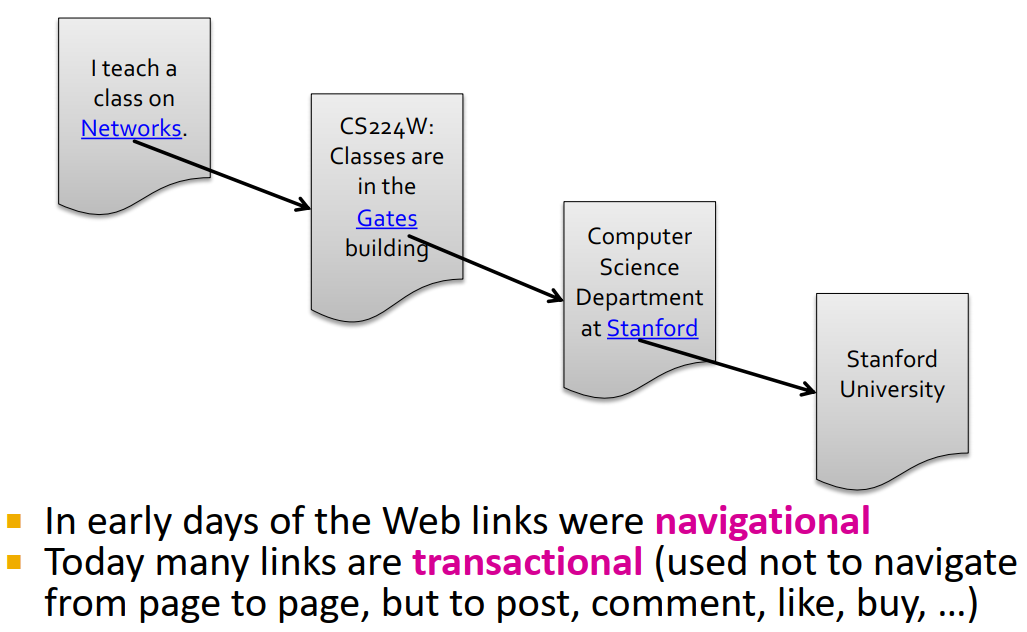4. 将网页看作有向图，以链接指向作为边的方向（这个网页/节点能直接跳转到的网页就作为其下一个节点successor）：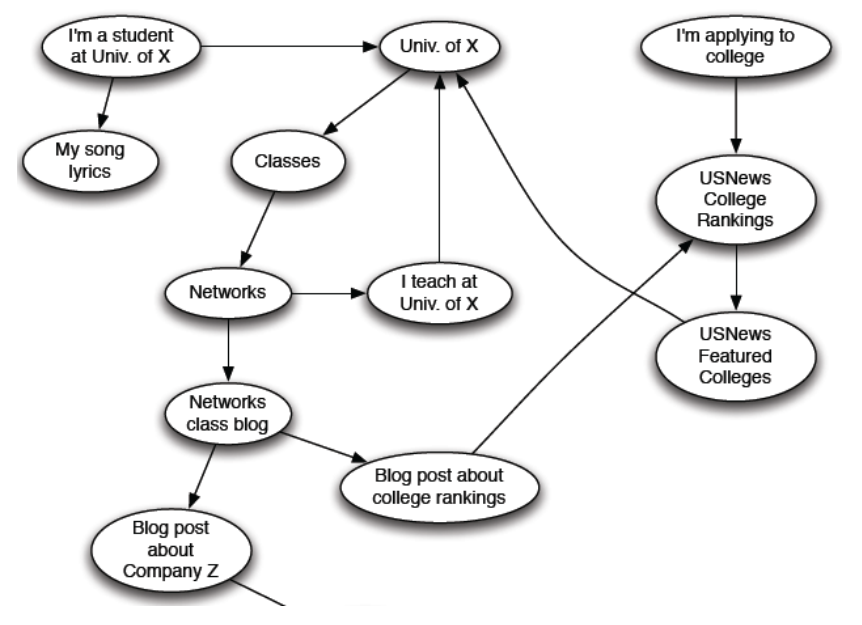5. 其他可表现为有向图形式的信息网络示例：论文引用，百科全书中词条间的互相引用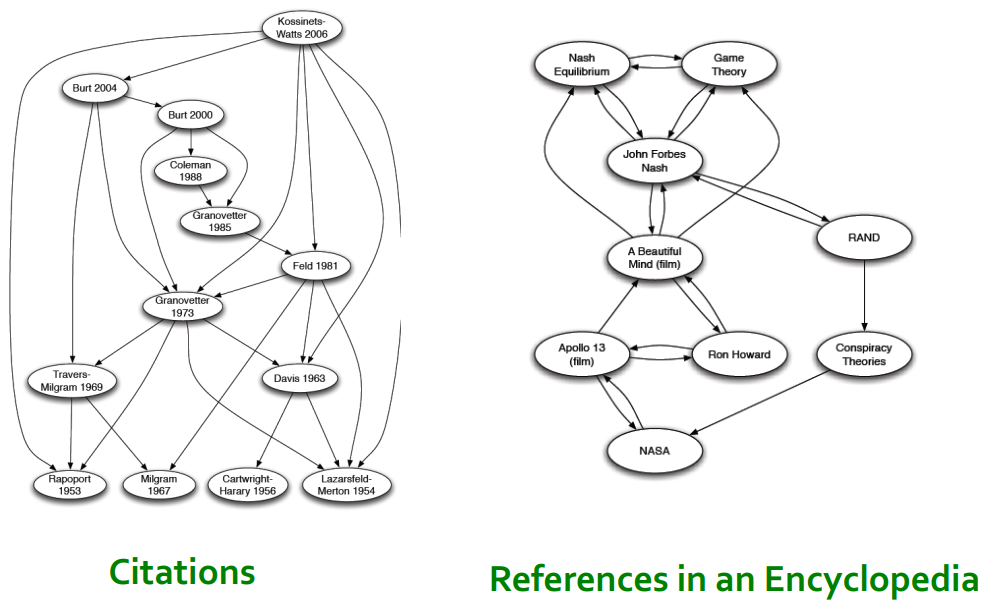6. 在图中，我们想要定义节点的重要性importance，通过网络图链接结构来为网页按重要性分级rank。以下将介绍3种用以计算图中节点重要性的方法：
1. PageRank
2. Personalized PageRank (PPR)
3. Random Walk with Restarts (RWR)
7. 衡量节点重要性：认为一个节点的链接越多，那么这个节点越重要。
这就成了一个递归recursive的问题——要计算一个节点的重要性就要先计算其predecessors的重要性，计算这些predecessors的重要性又要先计算它们predecessors的重要性……

## 2.1 PageRank: The “Flow” Model

1. 链接权重与其source page的重要性成正比例
2. 如果网页 i i 的重要性是 r i r_i ，有 d i d_i 个out-links，那么每个边的权重就是 r i / d i r_i/d_i
3. 网页 j j 的重要性 r j r_j 是其in-links上权重的总和4. 从而得到对节点 j j 的级别 r j r_j 的定义： r j = ∑ i → j r i d i r_j=\sum\limits_{i\rightarrow j}\frac{r_i}{d_i} d i d_i 是节点 i i 的出度）
5. 以这个1839年的网络为例：我们就可以得到这样的"flow" equations:
r y = r y / 2 + r a / 2 r_y=r_y/2+r_a/2
r a = r y / 2 + r m r_a=r_y/2+r_m
r m = r a / 2 r_m=r_a/2
6. 在直觉上我们好像可以用高斯消元法Gaussian elimination来解这个线性方程组，但这种方法不scalable。所以我们寻找更scalable的矩阵形式解法。

## 2.2 PageRank: Matrix Formulation

1. 建立随机邻接矩阵stochastic adjacency matrix M：网页 j j d j d_j 条out-links，如果 j → i j\rightarrow i M i j = 1 d j M_{ij}=\frac{1}{d_j}
M是列随机矩阵column stochastic matrix（列和为1）3
M的第 j j 列可以视作 j j 在邻居节点上的概率分布2. rank vector r r : 每个网页 i i 的重要性得分 r i r_i
∑ i r i = 1 \sum_i\mathbf{r}_i=1 （所以 r r 也可被视为是网络中所有节点的概率分布）
3. flow equations可以被写成： r = M ⋅ r \mathbf{r}=M\cdot \mathbf{r}
回忆一下原公式： r j = ∑ i → j r i d i r_j=\sum\limits_{i\rightarrow j}\frac{r_i}{d_i} （其实感觉从这个公式推到上个公式应该还是比较直觉的， M M 的第 j j 行被指向的节点对应的列的元素就是 1 / d i 1/d_i ，该列对应的是 r i \mathbf{r}_i ，加总起来就得到上个公式）
4. flow equation和矩阵对比的举例：## 2.3 Connection to Random Walk

1. 假想一个web surfer的随机游走过程，在 t 时间在网页 i i 上，在 t+1 时间从 i i 的out-links中随机选一条游走。如此重复过程。
向量 p ( t ) \mathbf{p}(t) 的第 i i 个坐标是 t 时间web surfer在网页 i i 的概率，因此向量 p ( t ) \mathbf{p}(t) 是网页间的概率分布向量。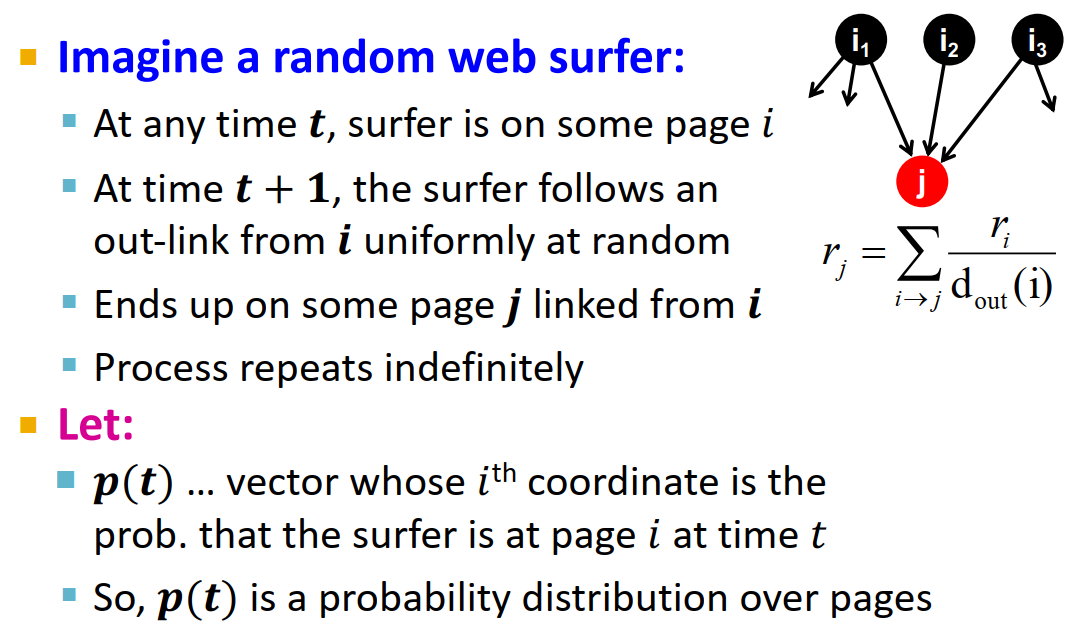2. 平稳分布stationary distribution
p ( t + 1 ) = M ⋅ p ( t ) \mathbf{p}(t+1)=M\cdot \mathbf{p}(t) （M是web surfer的转移概率，这个公式的逻辑感觉和 r = M ⋅ r \mathbf{r}=M\cdot \mathbf{r} 其实类似）
如果达到这种条件，即下一时刻的概率分布向量与上一时刻的相同： p ( t + 1 ) = M ⋅ p ( t ) = p ( t ) \mathbf{p}(t+1)=M\cdot \mathbf{p}(t)=\mathbf{p}(t) p ( t ) \mathbf{p}(t) 是随机游走的stationary distribution
r = M ⋅ r \mathbf{r}=M\cdot \mathbf{r} ，所以 r \mathbf{r} 是随机游走的stationary distribution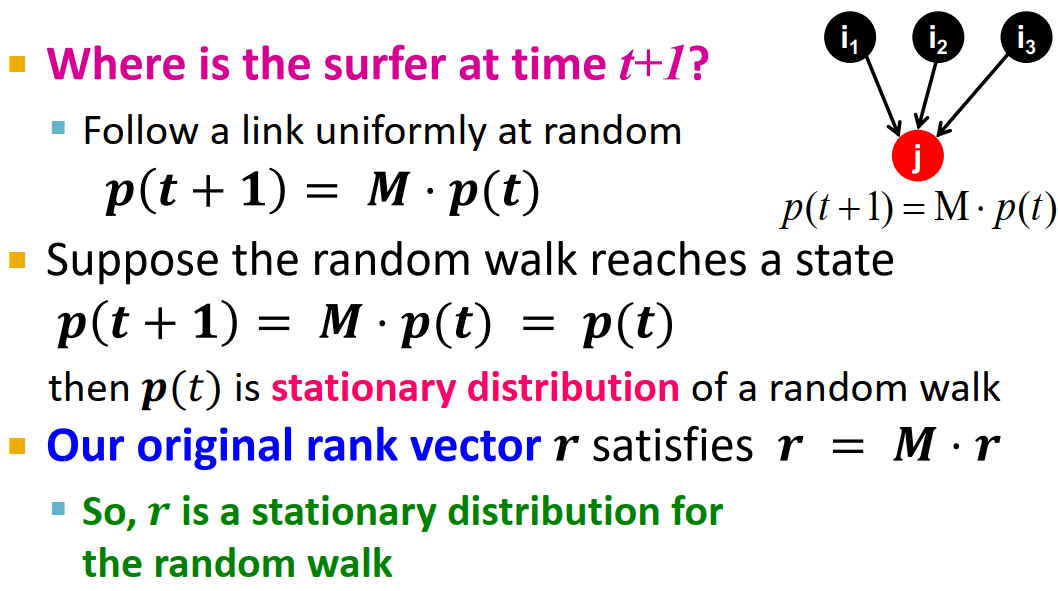这种做法将PageRank与随机游走概念进行了联合

## 2.4 Eigenvector Formulation

1. 无向图的邻接矩阵的特征向量是节点特征eigenvector centrality4，而PageRank定义在有向图的随机邻接矩阵上。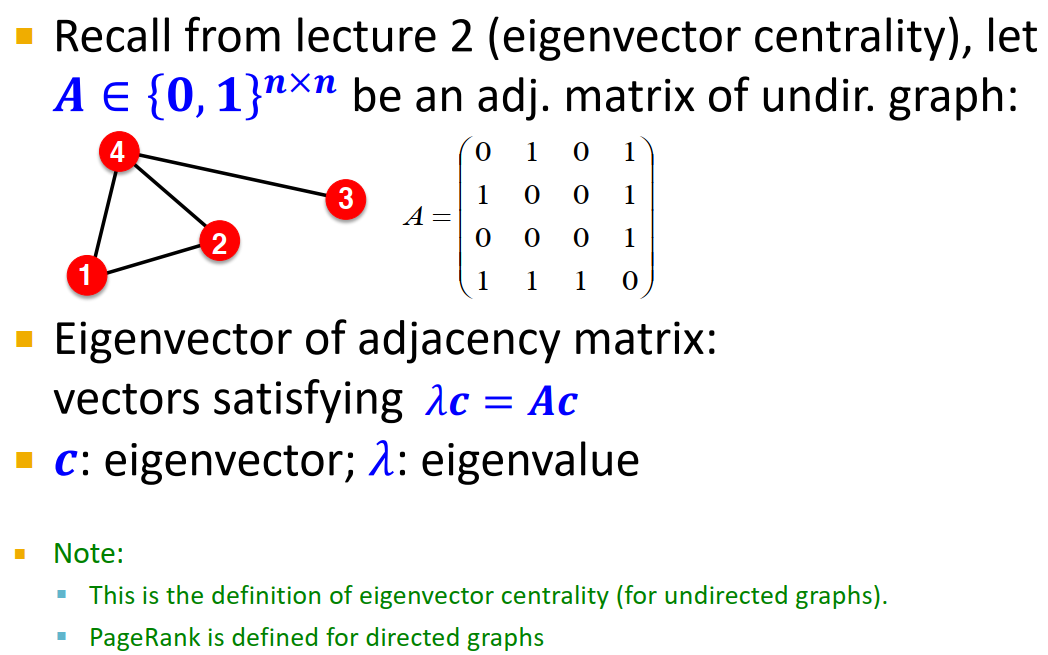2. 1 ⋅ r = M ⋅ r 1\cdot\mathbf{r}=M\cdot\mathbf{r}
rank vector r \mathbf{r} 是随机邻接矩阵M的特征向量，对应特征值为1。
从任一向量 u \mathbf{u} 开始，极限 M ( M ( . . . M ( M u ) ) ) M(M(...M(M\mathbf{u}))) 是web surfer的长期分布，PageRank=极限分布=M的principal eigenvector5
根据这个思路，我们就能找到PageRank的求解方式：power iteration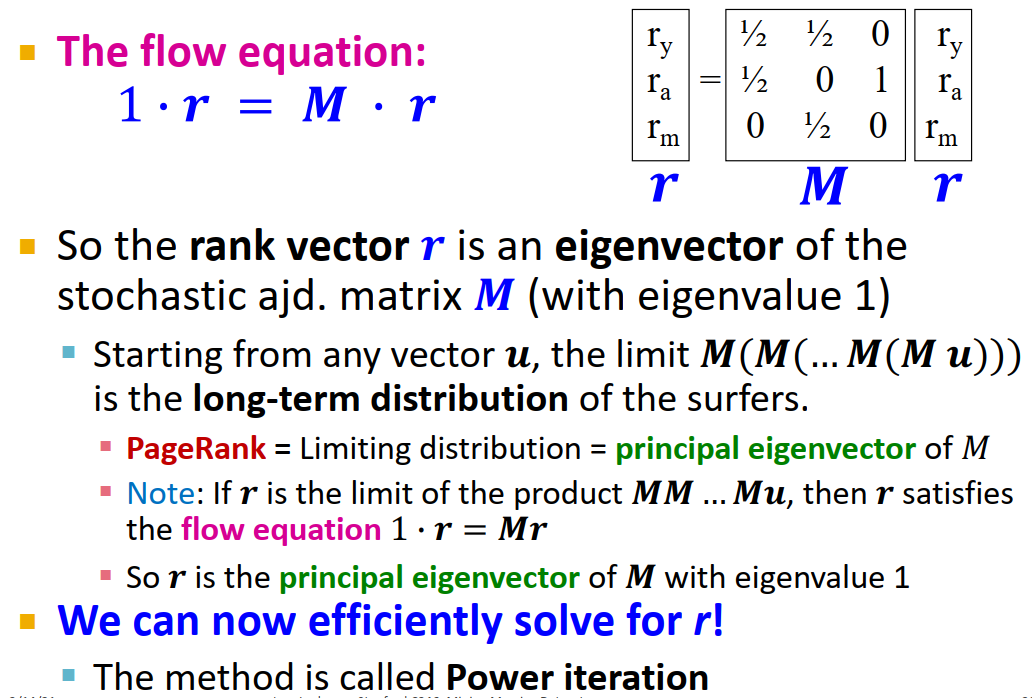limit极限
limiting distribution极限分布
6
相当于是random surfer一直随机游走，直至收敛，到达稳定状态。
这个M的叠乘可以让人联想到Katz index叠乘邻接矩阵A7
相比高斯消元法，power iteration是一种scalable的求解PageRank方法。
3. PageRank总结
1. 通过网络链接结构衡量图中节点的重要性
2. 用随机邻接矩阵M建立web surfer随机游走模型
3. PageRank解方程： r = M ⋅ r \mathbf{r}=M\cdot \mathbf{r}
r \mathbf{r} 可被视作M的principle eigenvector，也可被视作图中随机游走的stationary distribution

# 3. PageRank: How to solve?

1. power iteration method 幂迭代法
初始化： r 0 = [ 1 / N , . . . , 1 / N ] T \mathbf{r}^0=[1/N,...,1/N]^T
迭代： r ( t + 1 ) = M ⋅ r t \mathbf{r}^{(t+1)}=M\cdot\mathbf{r}^t 直至 ∣ r ( t + 1 ) − r t ∣ 1 < ϵ |\mathbf{r}^{(t+1)}-\mathbf{r}^t|_1<\epsilon
约50次迭代即可逼近极限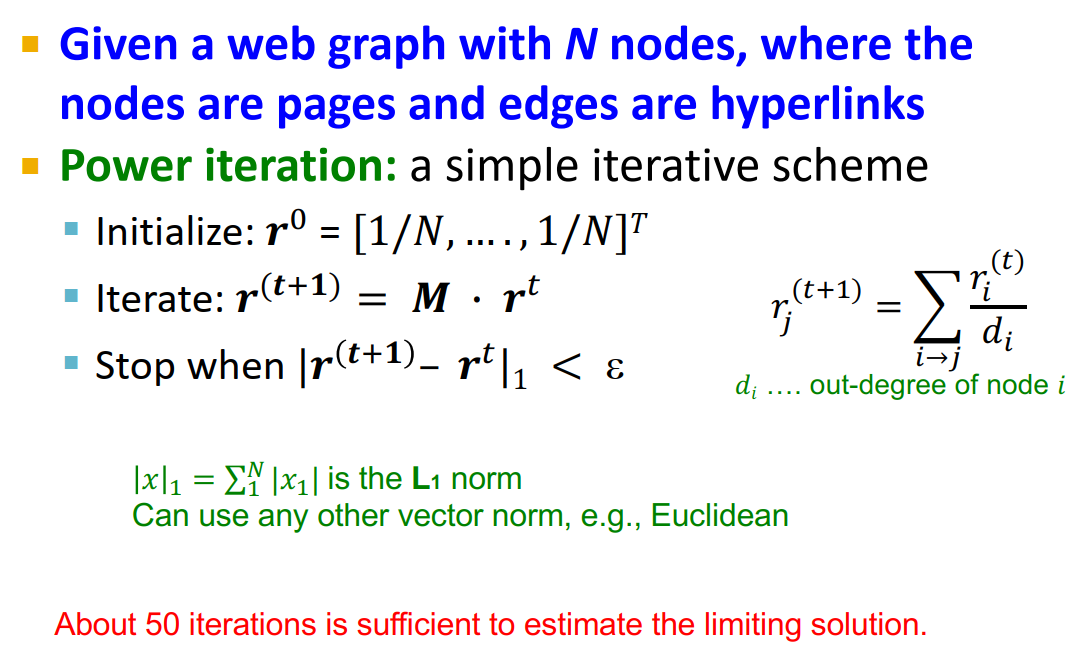2. power iteration示例：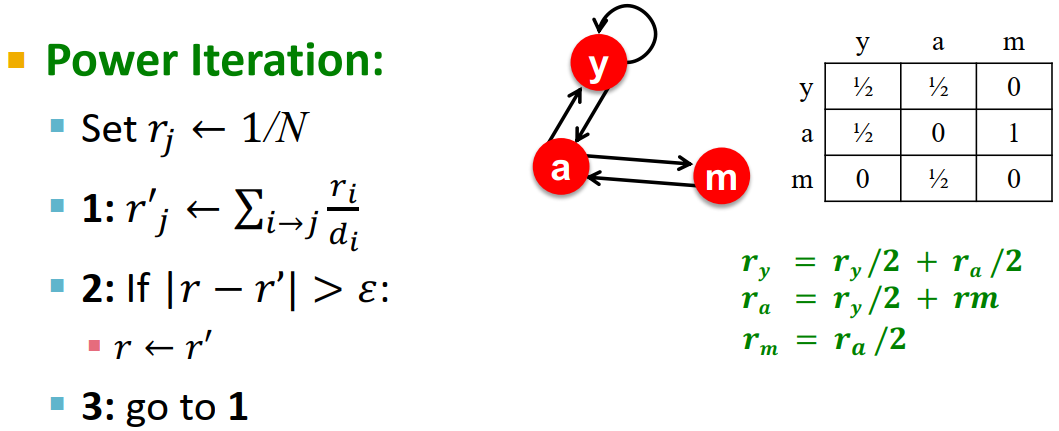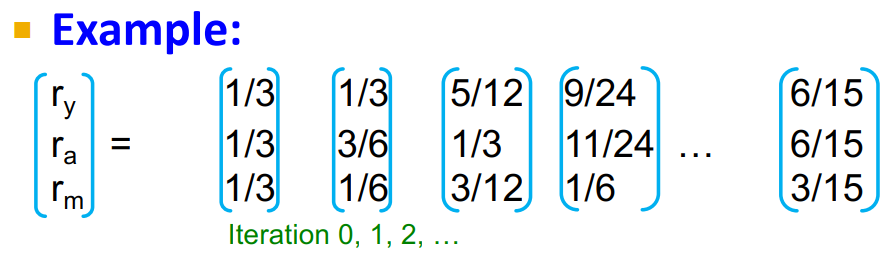3. PageRank的问题及其解决方案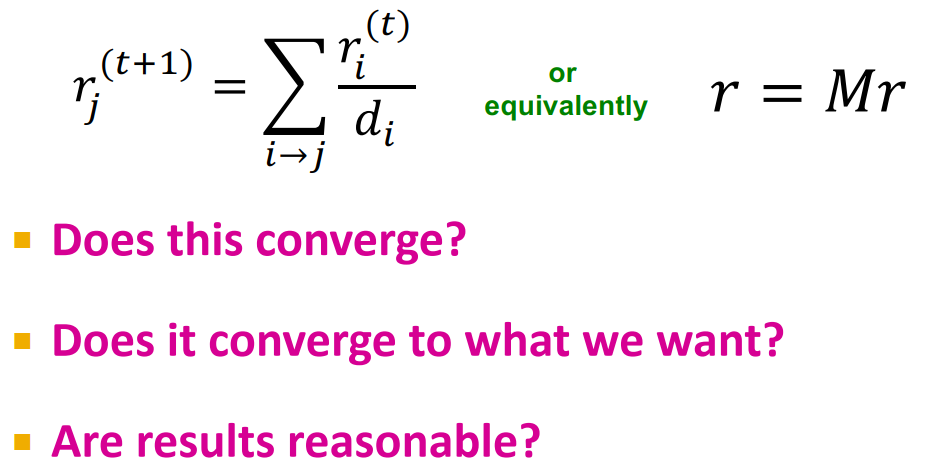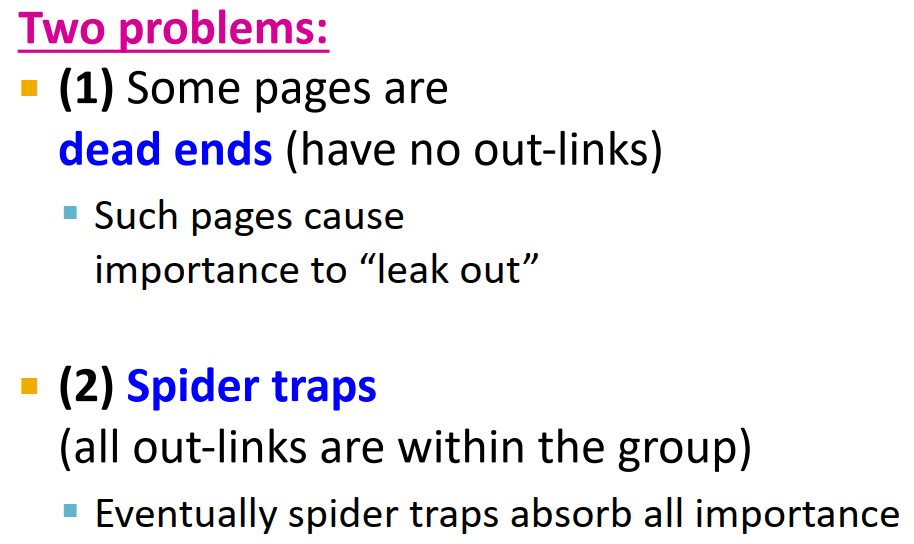1. spider trap：所有出边都在一个节点组内，会吸收所有重要性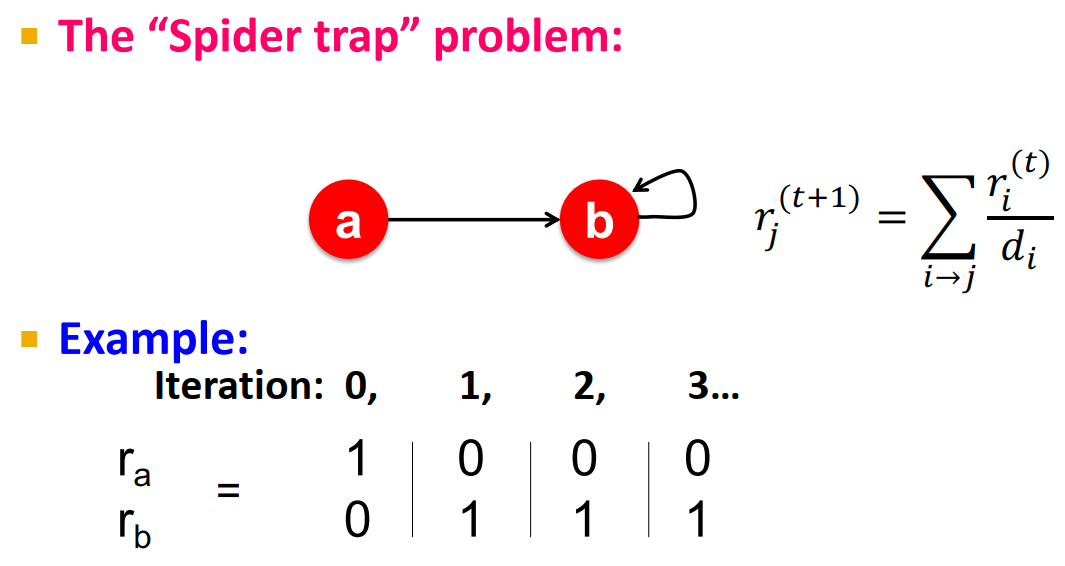random surfer会在圈子里打转，出不去
2. dead end：没有出边，造成重要性泄露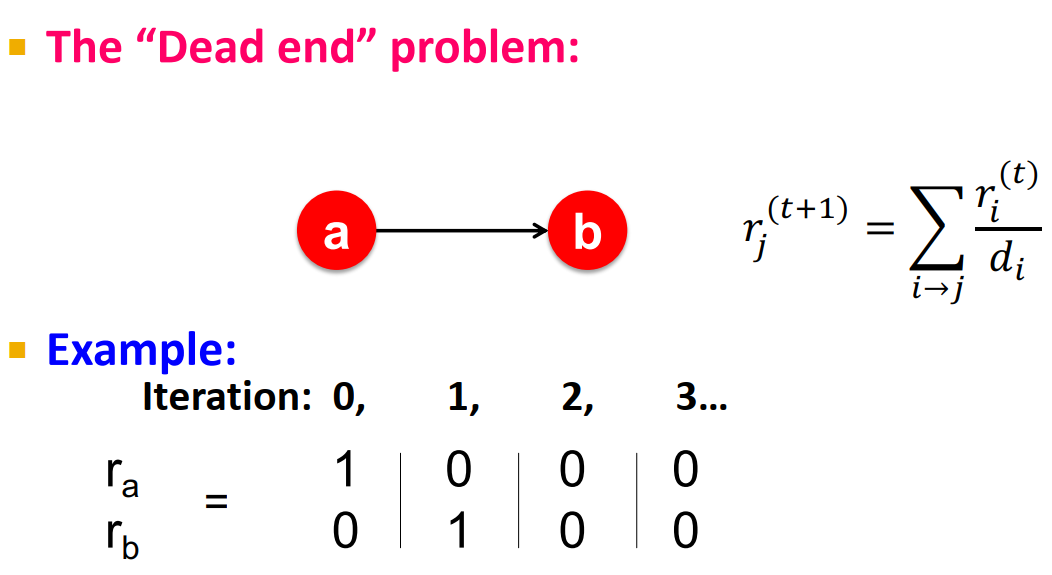random surfer无处可去，迷路重要性直接跳崖……
3. spider traps解决方案：random jumps or teleports（teleport（通常见于科幻作品）（被）远距离传送
random surfer每一步以概率 β \beta 随机选择一条链接（用M），以概率 1 − β 1-\beta 随机跳到一个网页上（ β \beta 一般在0.8-0.9之间）
这样surfer就会在几步后跳出spider trap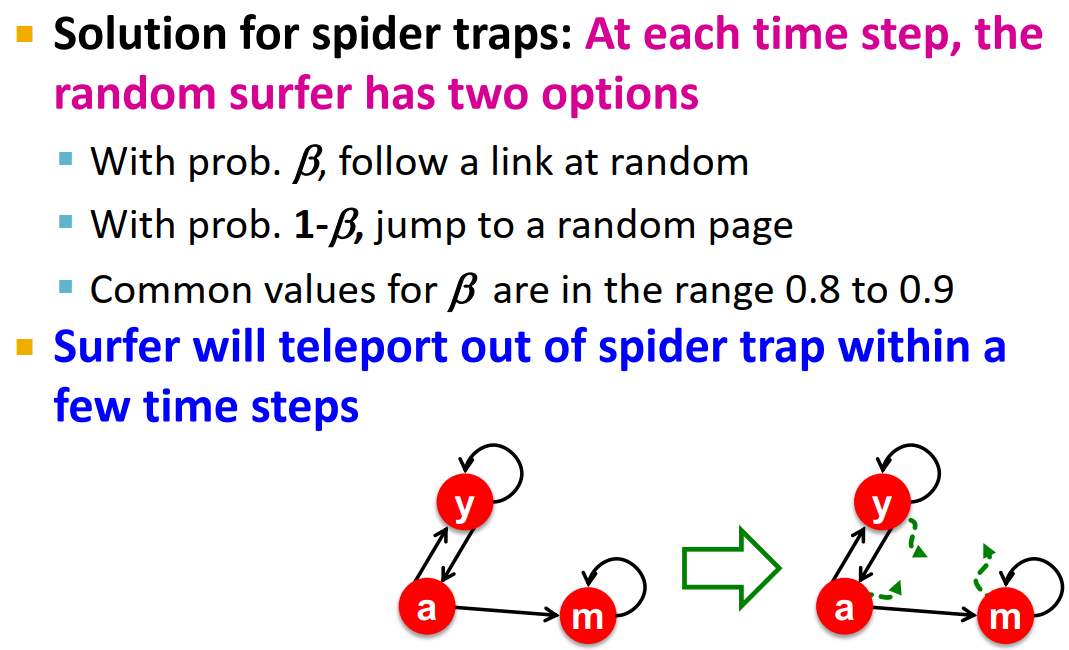4. dead ends解决方案：random jumps or teleports
从dead-ends出发的web surfer随机跳到任意网页（相当于从dead end出发，向所有节点连了一条边）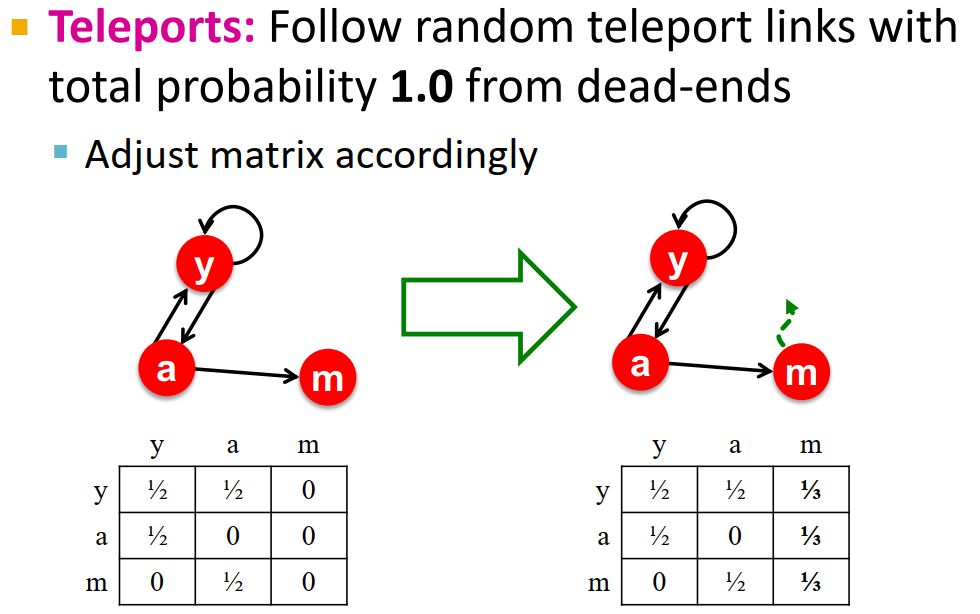5. spider-traps在数学上不是个问题，但是无法得到我们想要的PageRank结果；因此要在有限步内跳出spider traps。6. 整体解决方案：random teleport
random surfer每一步以概率 β \beta 随机选择一条链接（M），以概率 1 − β 1-\beta 随机跳到一个网页上。
整体公式为： r j = ∑ i → j β r i d i + ( 1 − β ) 1 N r_j=\sum\limits_{i\rightarrow j}\beta\frac{r_i}{d_i}+(1-\beta)\frac{1}{N} d i d_i 是节点 i i 的出度）
M需要没有dead ends，可以通过直接去除所有dead ends或显式将dead ends跳到随机节点的概率设置到总和为1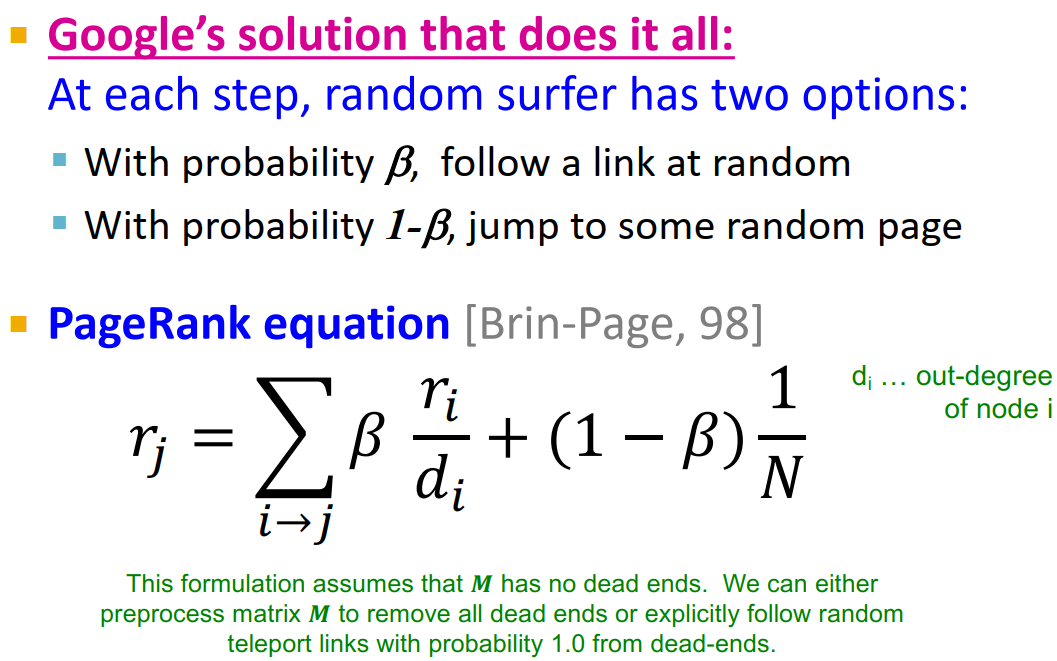7. The Google Matrix G
G = β M + ( 1 − β ) [ 1 N ] N × N G=\beta M+(1-\beta)\left[\frac{1}{N}\right]_{N\times N}
[ 1 N ] N × N \left[\frac{1}{N}\right]_{N\times N} 是每个元素都是 1 N \frac{1}{N} N × N N\times N 的矩阵）

现在 r = G ⋅ r \mathbf{r}=G\cdot\mathbf{r} 又是一个迭代问题，power iteration方法仍然可用
β \beta 在实践中一般用0.8或0.9（平均5步跳出一次）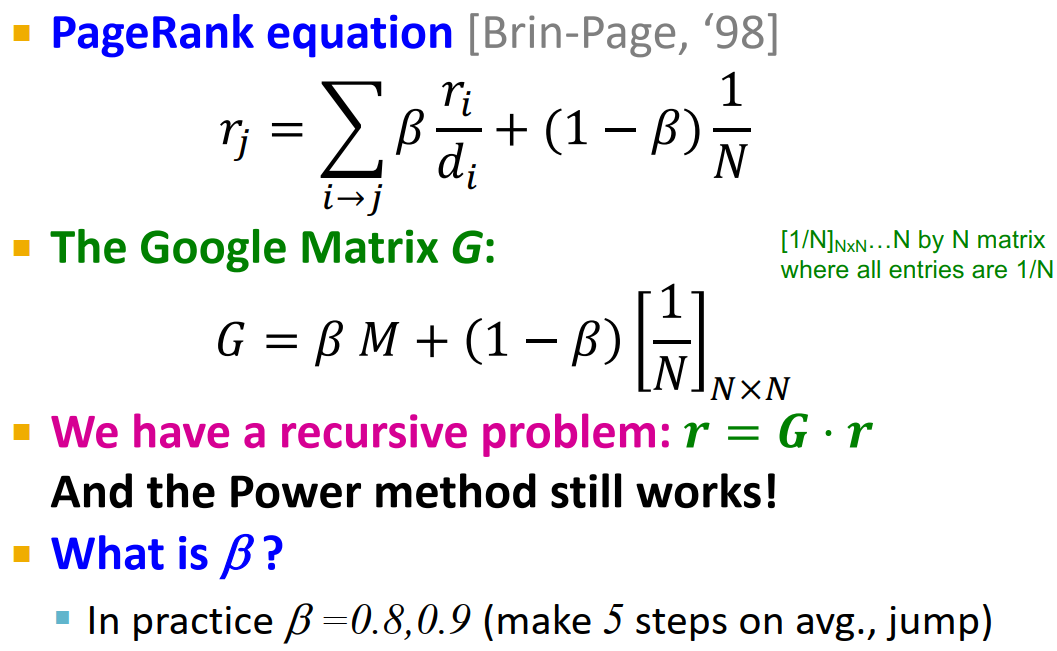注意：在本节课中random walk只是一种直觉假设，我们没有真的模拟random walk
8. random teleports举例：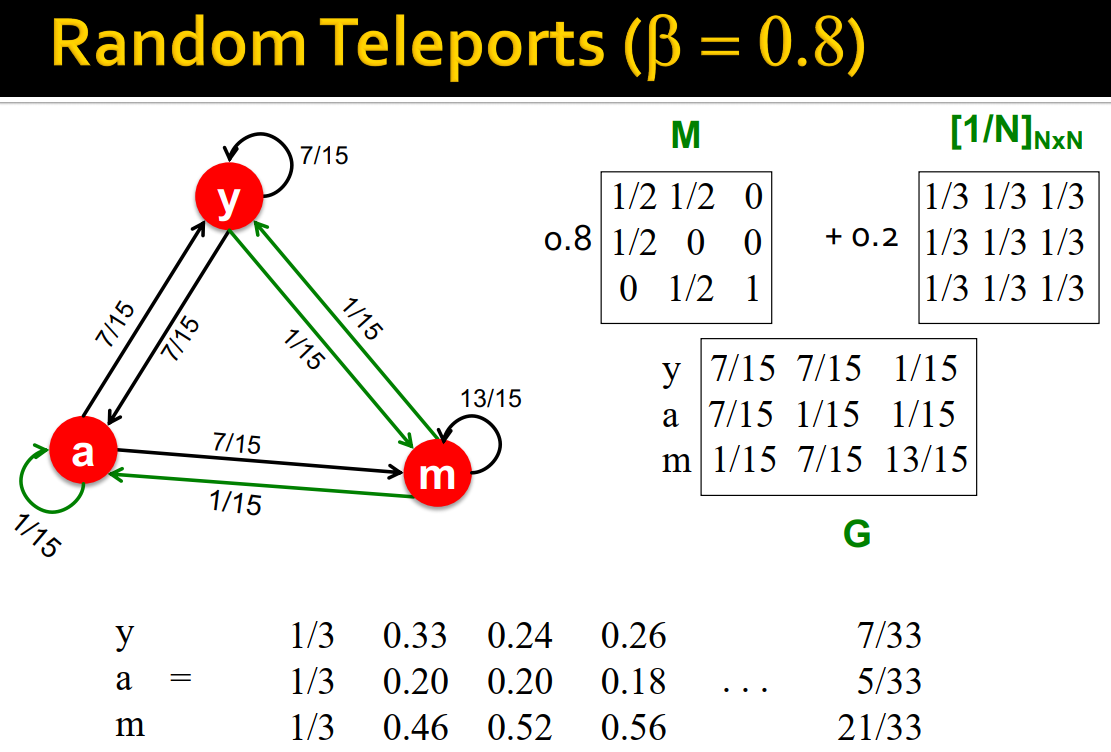M是个spider trap，所以加上random teleport links
G也是一个转移概率矩阵
4. PageRank结果示例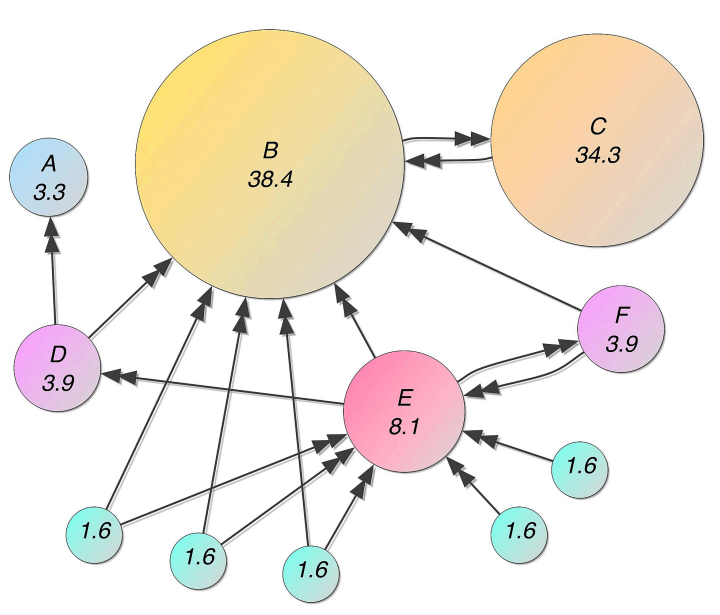5. PageRank求解部分总结：
1. 用power iteration方法求解 r = G ⋅ r \mathbf{r}=G\cdot\mathbf{r} （G是随机邻接矩阵）
2. 用random uniform teleporation解决dead-ends和spider-traps问题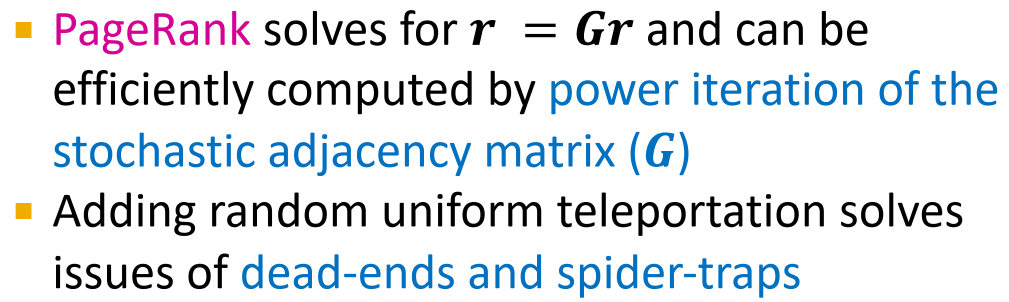# 4. Random Walk with Restarts & Personalized PageRank

1. 举例：推荐问题（一个由user和item两种节点组成的bipartite graph）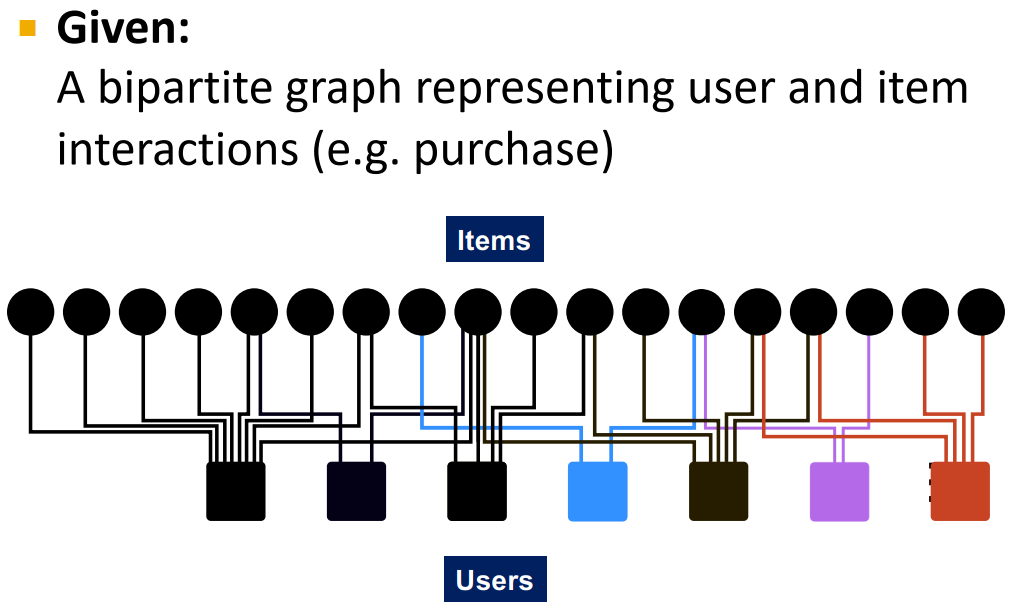2. Bipartite User-Item Graph
求解目标：图节点间相似性（针对与item Q交互的user，应该给他推荐什么别的item？）
可以直觉地想到，如果item Q和P都与相似user进行过交互，我们就应该推荐Q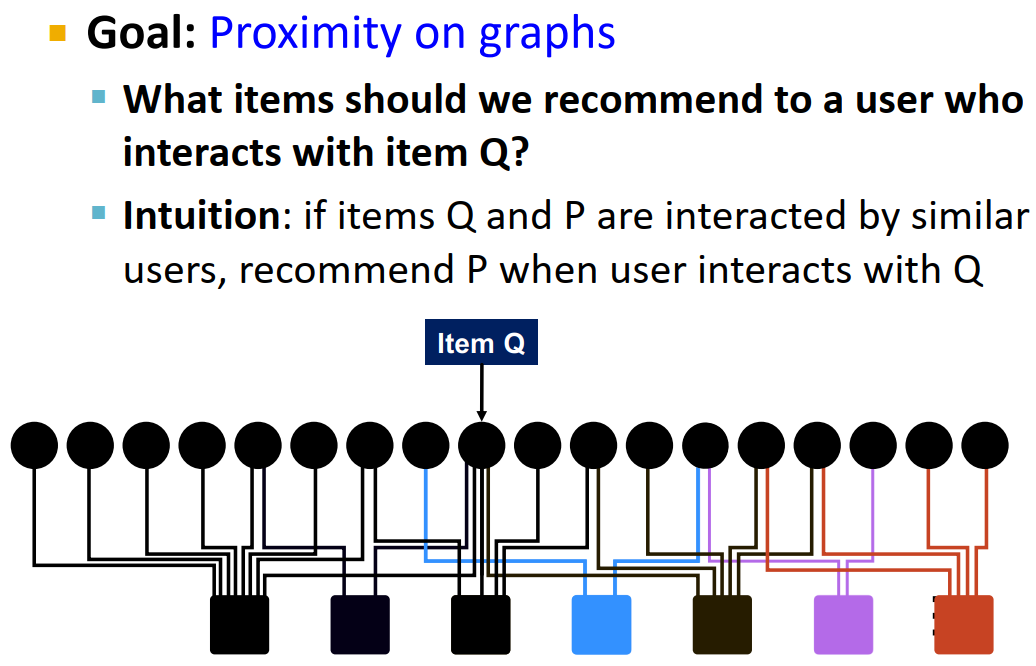但是我们如何量化这个相似性呢？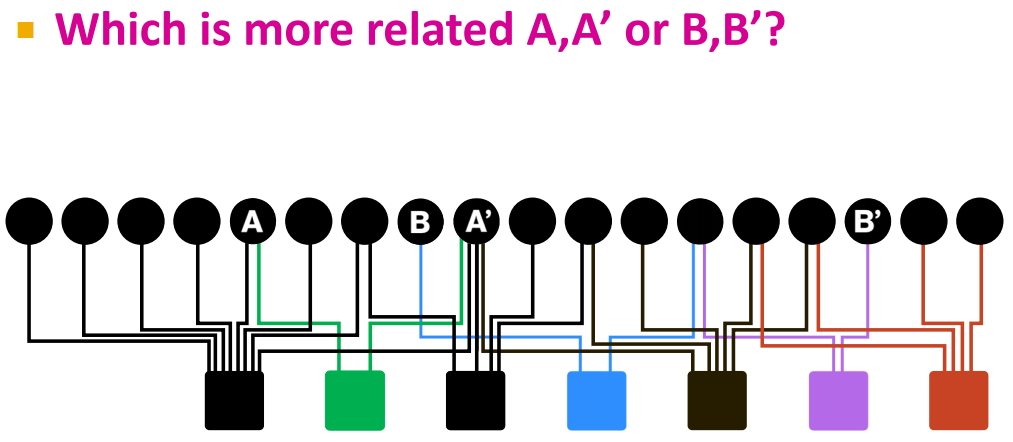3. 衡量节点相似性的问题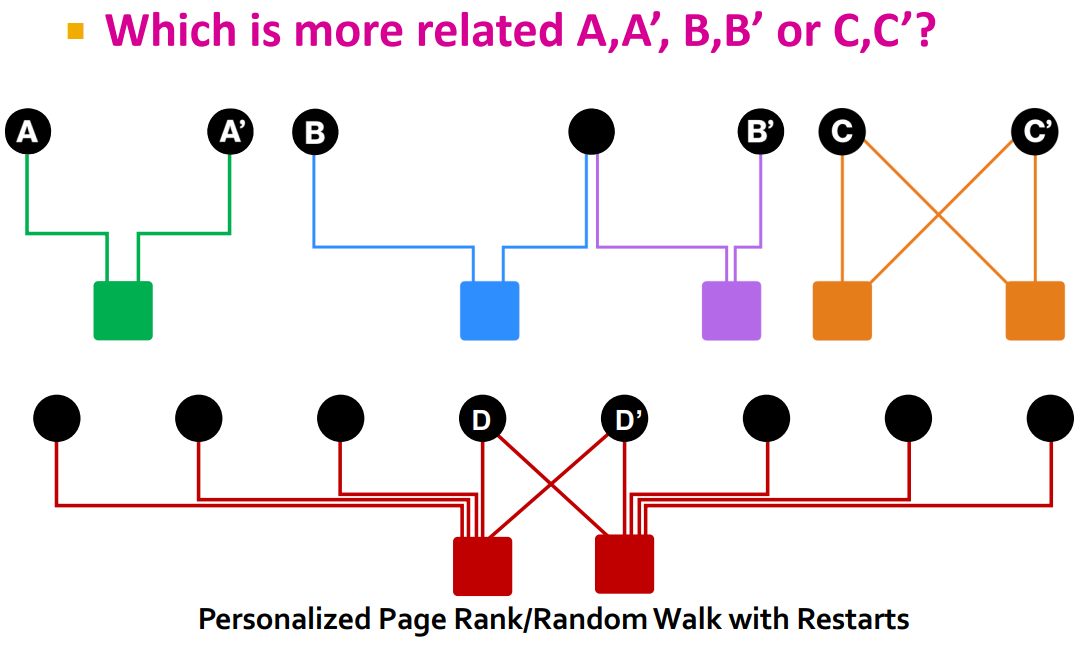A A’比B B’近可以因为它们之间距离较短；但是A A’和C C’距离相等，C C’却有着共同用户，这又如何比较呢？如果引入shared neighbors作为标准，D D’和C C’有相同的share neighbors，但是D D’的共同用户之间的相似性却很低，这又如何衡量呢？
4. 图上的相似性：Random Walks with Restarts
PageRank用重要性来给节点评级，随机跳到网络中的任何一个节点
Personalized PageRank衡量节点与teleport nodes S \mathbf{S} 中的节点的相似性
用于衡量其他item与item Q的相似性：Random Walks with Restarts只能跳到起始节点： S = { Q } \mathbf{S}=\{\mathbf{Q}\}
（所以RWR算是PPR的特例吧？虽然我百度了一下发现没这种说法……但是本课程的意思应该是算）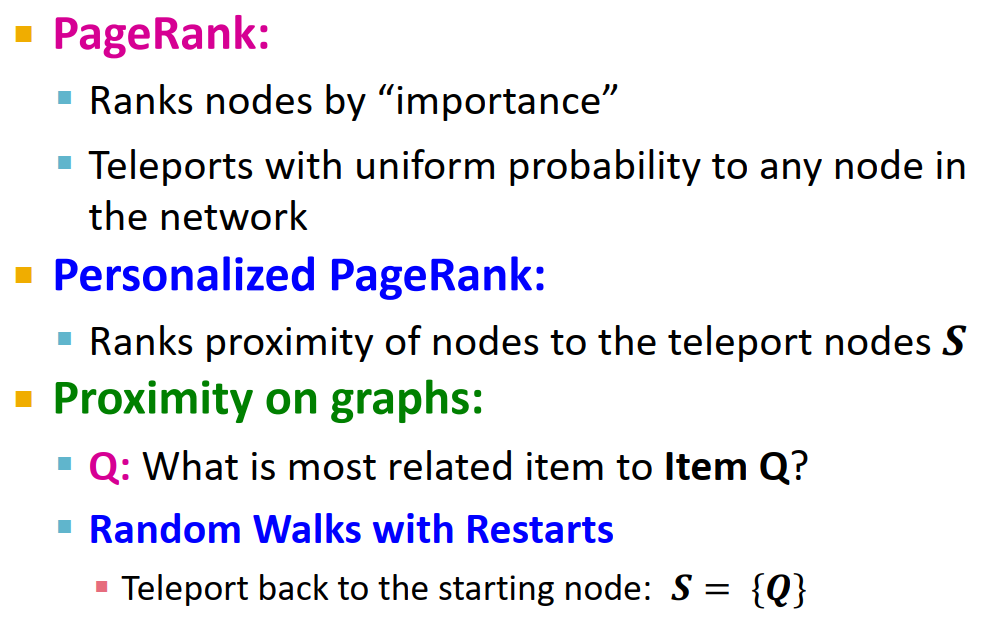PageRank, PPR和RWR是同一种算法，只在teleport set上有区别
5. Random Walks
每个节点都有重要性，在其边上均匀分布，传播到邻居节点。
对 query_nodes 模拟随机游走：
1. 随机游走到一个邻居，记录走到这个节点的次数（visit count）
2. 以 alpha 概率从 query_nodes 中某点重启
3. 结束随机游走后，visit count最高的节点就与 query_nodes 具体最高的相似性（直觉上这就是 query_nodes 最容易走到、最近的点了）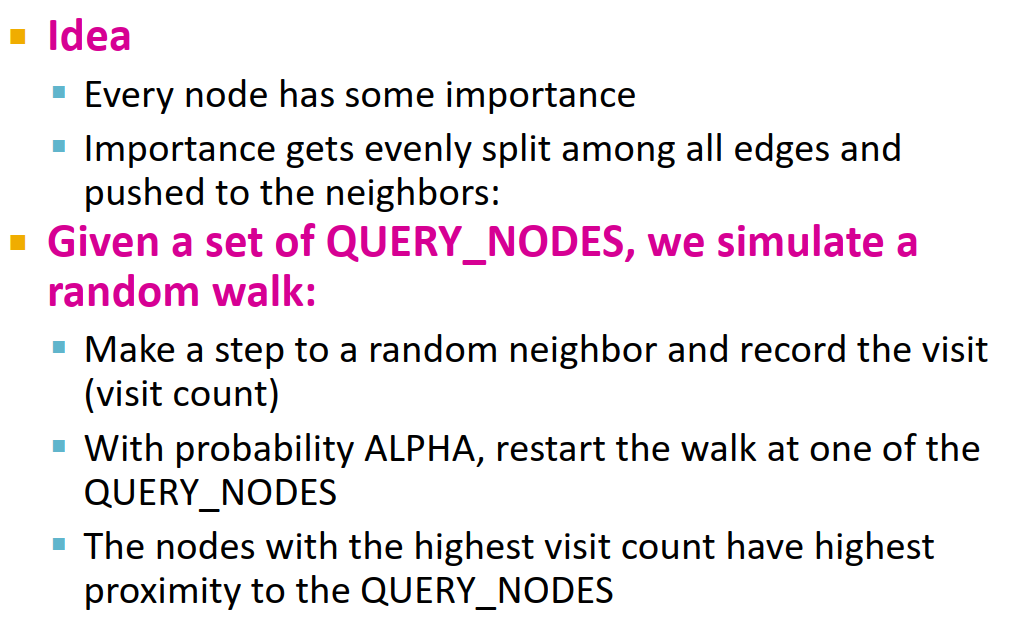6. 以 Q 作为示例：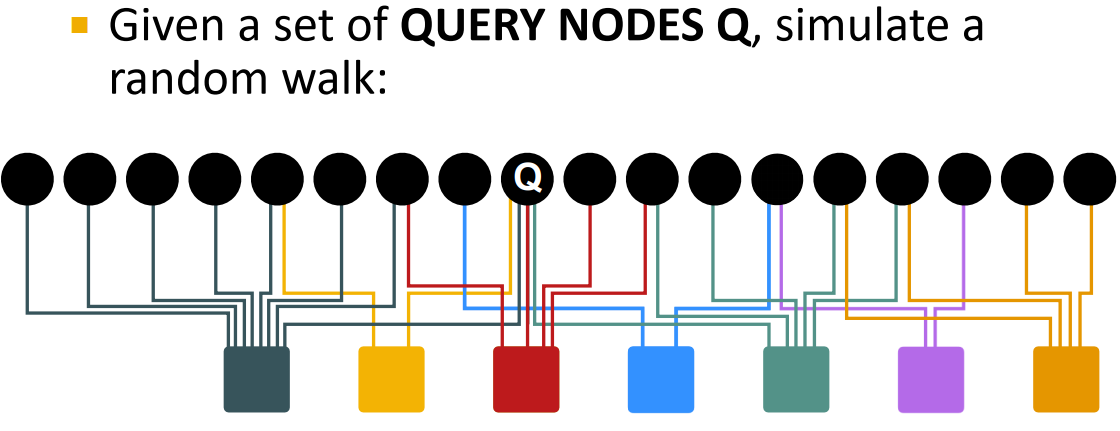算法伪代码（从item随机游走到另一个item，记录visit_count；以一定概率返回query_nodes）：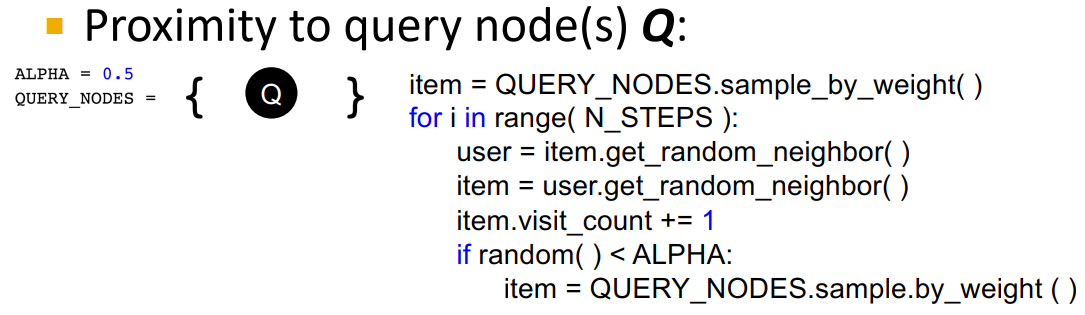结果示例：在示例中是模拟的random walk，但其实也可以用power iteration的方式来做

7. RWR的优点在于，这种相似性度量方式考虑到了网络的如下复杂情况：
1. multiple connections
2. multiple paths
3. direct and indirect connections
4. degree of the node8. 对不同PageRank变体的总结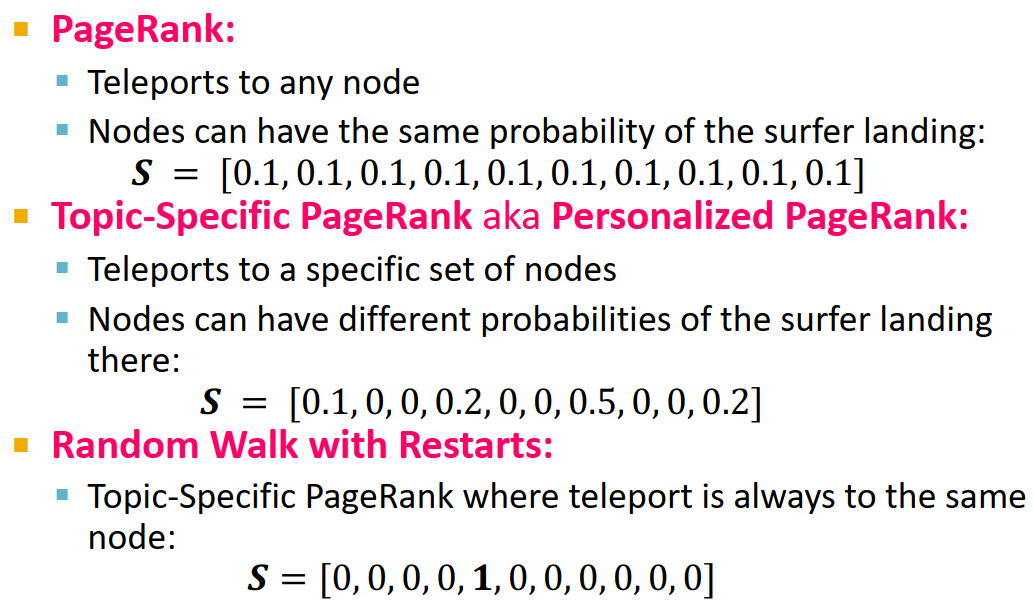9. 总结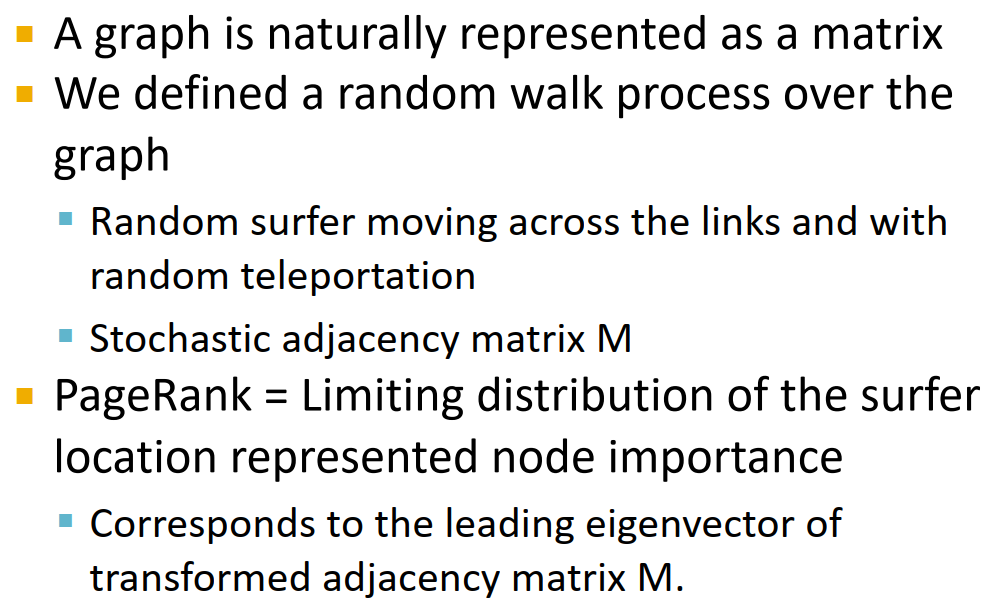# 5. Matrix Factorization and Node Embeddings

1. 回忆上一章8讲到的embedding lookup的encoder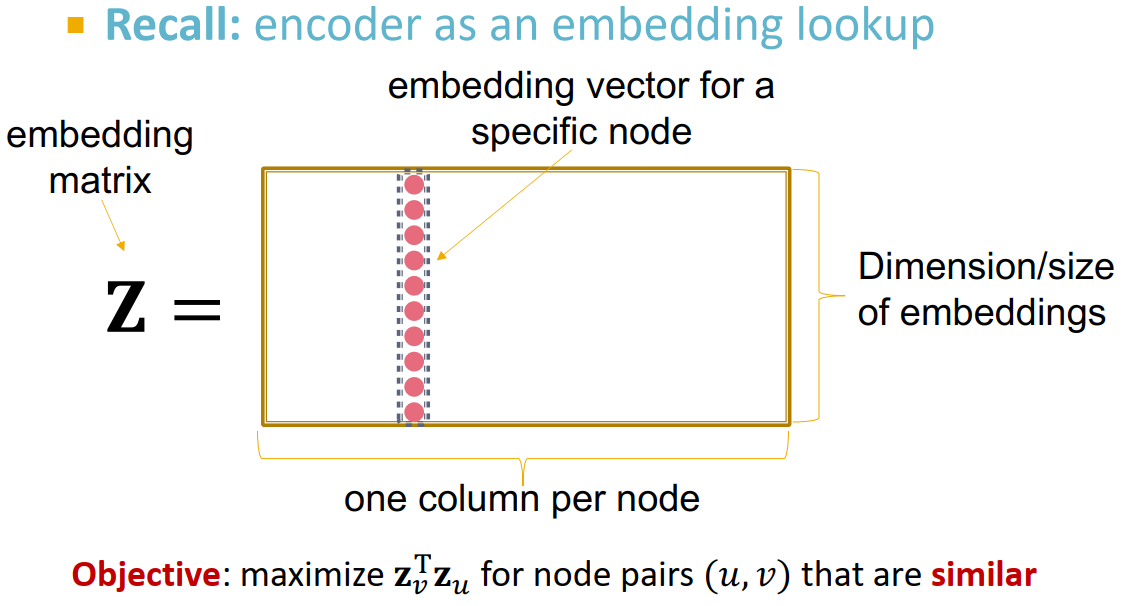2. 将节点嵌入视作矩阵分解：
假设有边的节点是相似节点，则 z v T z u = A u , v \mathbf{z}_v^T\mathbf{z}_u=A_{u,v} （A是邻接矩阵）(如有边连接，则节点嵌入相似性直接取到1)
Z T Z = A \mathbf{Z}^T\mathbf{Z}=A 感觉看图还挺直觉的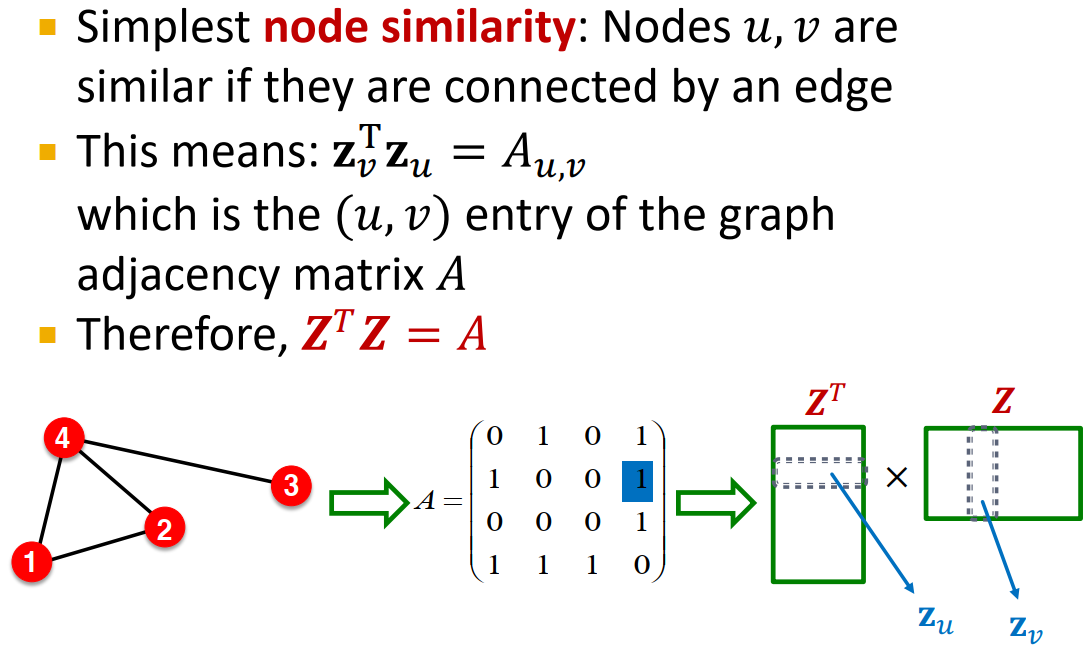3. 矩阵分解问题
节点表示向量维度远低于节点数。
如上一序号，将节点嵌入视作矩阵分解问题，严格的矩阵分解 A = Z T Z A=\mathbf{Z}^T\mathbf{Z} 很难实现（因为没有那么多维度来表示），因此通过最小化 A A Z T Z \mathbf{Z}^T\mathbf{Z} 间的L2距离（元素差的平方和）来近似目标。
在Lecture 38中我们使用softmax来代替L2距离完成目标9
所以用边连接来定义节点相似性的inner product decoder等同于A的矩阵分解问题。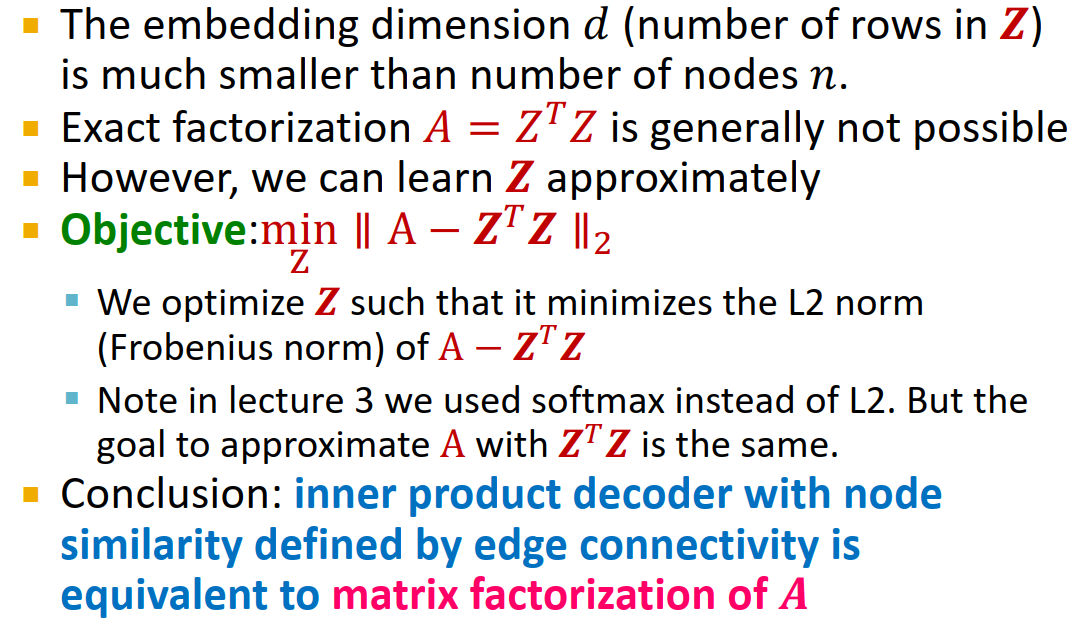4. 矩阵分解问题：基于random walk定义的相似性（这部分公式我完全没看懂，以后有缘看论文10
DeepWalk和node2vec有基于random walk定义的更复杂的节点相似性，但还是可以视作矩阵分解问题，不过矩阵变得更复杂了。（相当于是把上面的A给换了）

DeepWalk：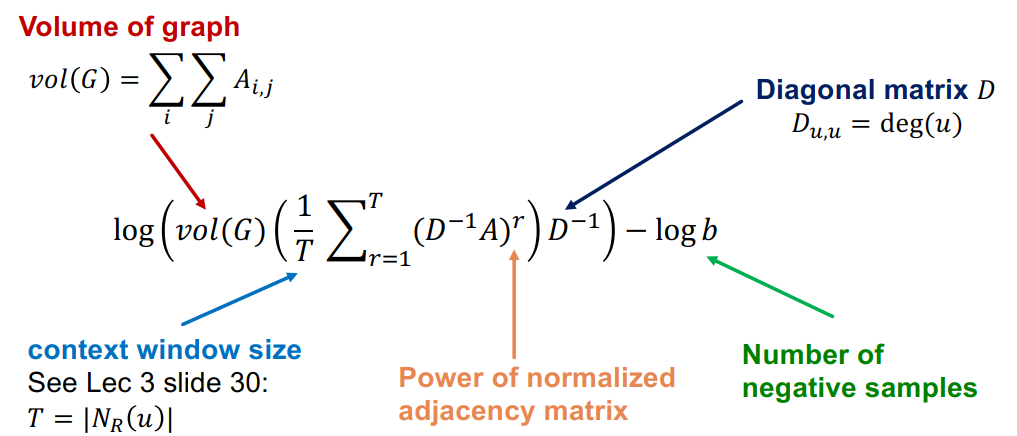albeit尽管
v o l ( G ) vol(G) 是2倍的边数

node2vec的更复杂
5. 通过矩阵分解和随机游走进行节点嵌入的限制
1. 无法获取不在训练集中的节点嵌入，每次新增节点都要对全部数据集重新计算嵌入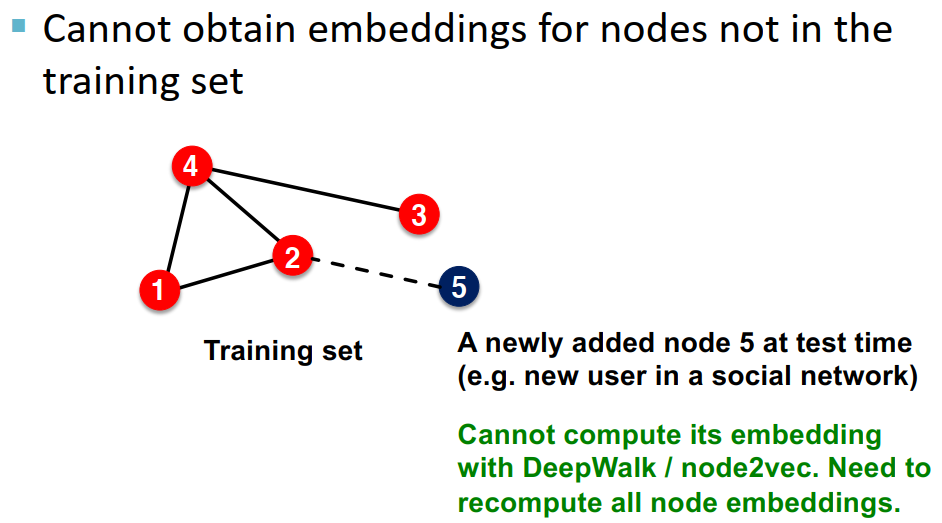（PageRank也可视作一维的节点嵌入）
2. 无法捕获结构相似性：比如图中节点1和节点11在结构上很相似，但是节点嵌入会差别很大（随机游走走不过去）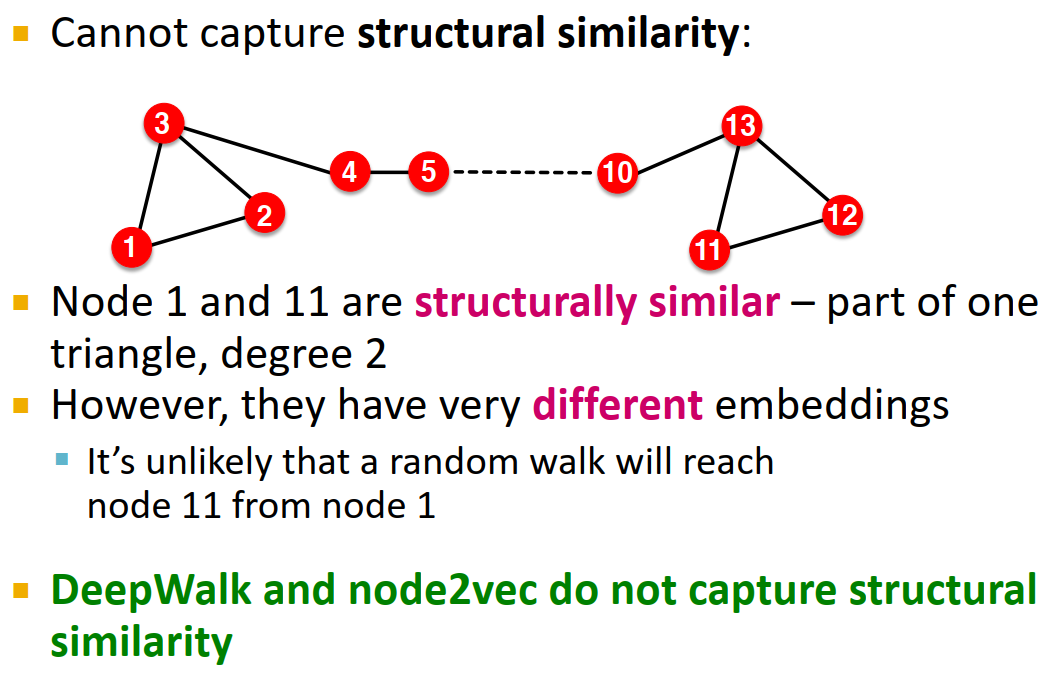（如果跑匿名随机游走的话，倒是能捕获到结构相似性）
3. 无法使用节点、边和图上的特征信息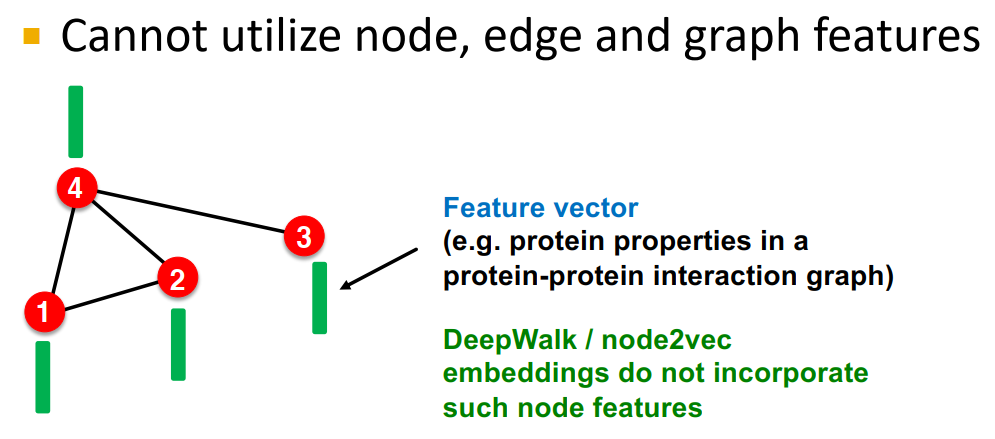4. 如何解决这些问题：Deep Representation Learning and Graph Neural Networks（后期讲解。我也将继续撰写相应笔记）

# 6. 本章总结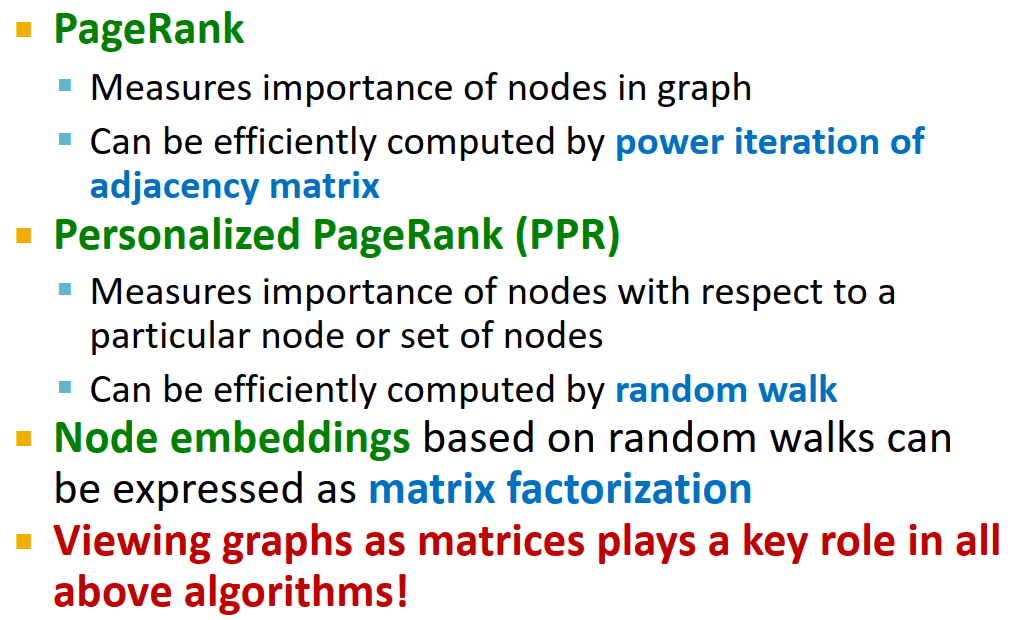# 7. 文中及脚注未提及的其他参考资料

1. CS224W-11 成就了谷歌的PageRank_公众号:图与推荐的博客-CSDN博客
2. 重启随机游走算法Random Walk with Restart (RWR) - 简书
3. 重启随机游走算法（RWR：Random Walk with Restart）_wangprince2017-CSDN博客
4. 个性化的PageRank和主题感知的PageRank_I am not a quitter.-CSDN博客

Brin S, Page L. The anatomy of a large-scale hypertextual web search engine[J]. Computer networks and ISDN systems, 1998, 30(1-7): 107-117.
Page L, Brin S, Motwani R, et al. The PageRank citation ranking: Bringing order to the web[R]. Stanford InfoLab, 1999. ↩︎

2. on the fly：不需要其他操作自动生成的，无需退出当前流程来做某件事，不经过某种额外步骤而直接进行某项活动，直接生效等意思。比如网页自动根据日期、用户之前看到的位置等信息向用户呈现的内容，就是on the fly
参考资料：
①如何优雅的翻译 on the fly ？ - 知乎
②What is on the fly? - Definition from WhatIs.com ↩︎

3. 我看了一下stochastic matrix这个概念，它涵盖了随机过程、马尔科夫性质……等一系列我搞不懂的高级知识。具体是个什么东西我以后再研究。
总之stochastic matrix是行或列和为1（column stochastic matrix就是列和为1）。
感觉上是个状态转移概率矩阵。
参考资料：随机矩阵（stochastic matrix） - huangshanshan - 博客园 ↩︎

4. 对eigenvector centrality的讲解可参考我之前写的博文：cs224w（图机器学习）2021冬季课程学习笔记2_诸神缄默不语的博客-CSDN博客 2. Traditional Feature-based Methods: Node ↩︎

5. 这个principal eigenvector是指什么我也没搞懂，我查了一下好像是对应最大特征值的特征向量（Linear algebra review 原话：The eigenvector corresponding to the eigenvalue of largest magnitude is called the principal eigenvector.），但是课程中又说是对应特征值为1的特征向量。
啥意思，到底是哪个？还是说M的最大特征值就是1？我没有搞懂。 ↩︎

6. 极限分布这个概念我百度了一下，也是马尔科夫链，概率论……之类的概念，我没搞懂。在课程中我就直接理解为概率分布随时间变化趋近于稳定后到达的状态了。 ↩︎

7. 对Katz index的讲解可参考我之前写的博文：cs224w（图机器学习）2021冬季课程学习笔记2_诸神缄默不语的博客-CSDN博客 3. Traditional Feature-based Methods: Link ↩︎

8. cs224w（图机器学习）2021冬季课程学习笔记3: Node Embeddings_诸神缄默不语的博客-CSDN博客 ↩︎ ↩︎

9. 其实这里我没搞懂。就是，我知道 Lecture 3 里面用softmax可以使相似节点的表示向量也相近，就和这里的L2距离想干的事一样，但是我不知道它能不能在数学上作为L2距离的代替，毕竟它干的事是使相似节点的表示向量的点乘最大化，这个L2距离干的事好像差不多但是我不知道能不能算是真的一样。 ↩︎

10. Network Embedding as Matrix Factorization: Unifying DeepWalk, LINE, PTE, and node2vec, WSDM 18 ↩︎### 低价透明### 金牌服务### 信息保密